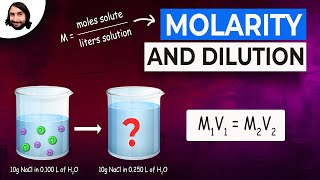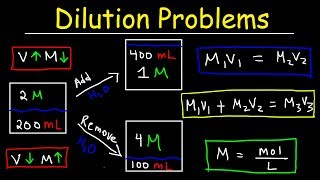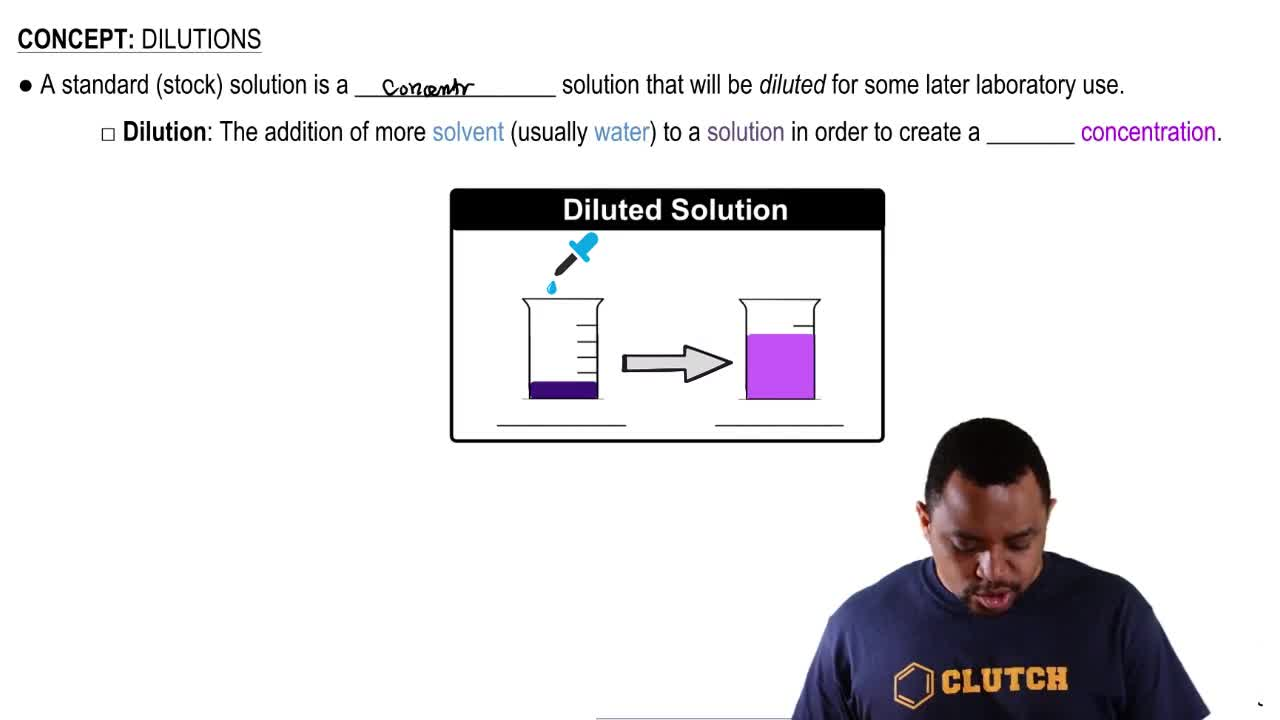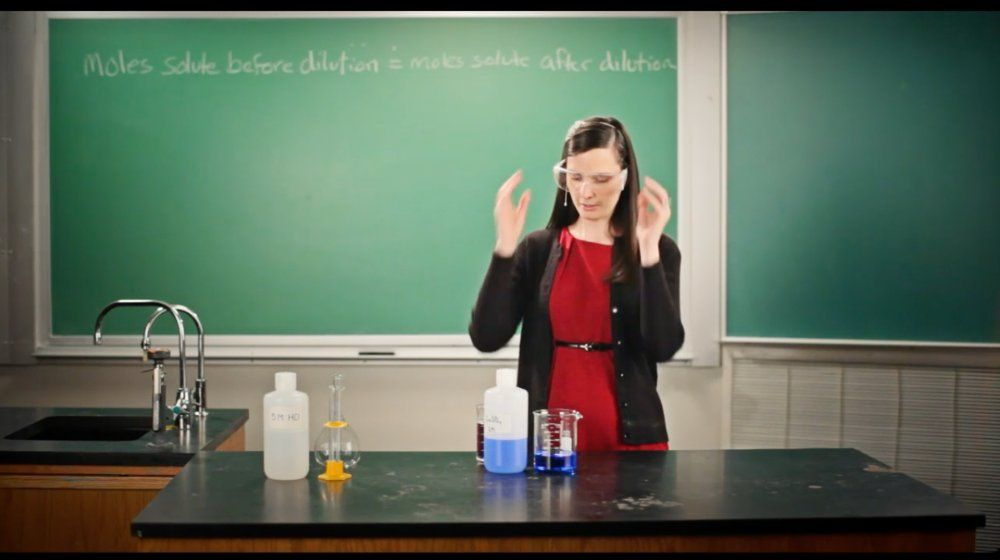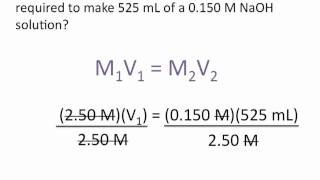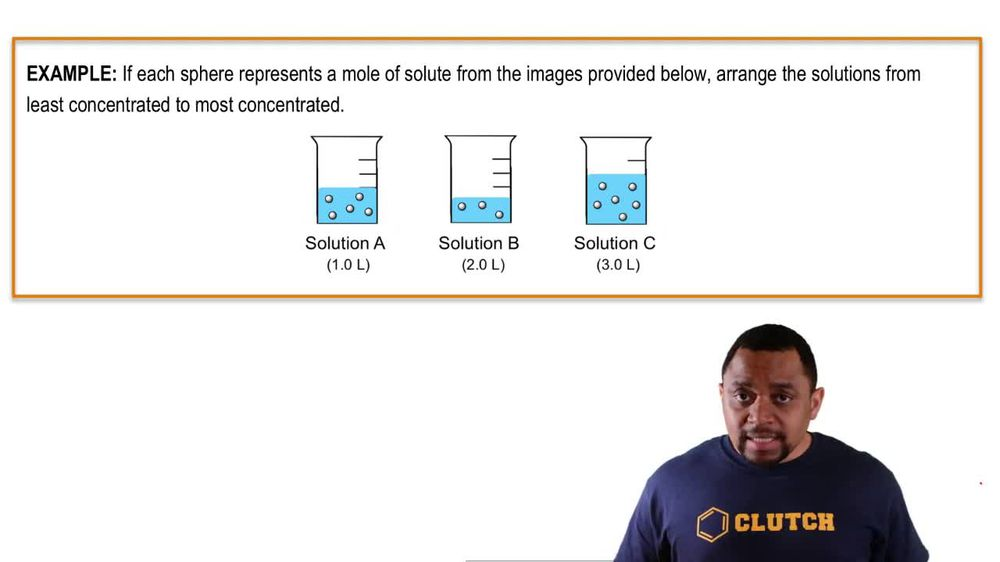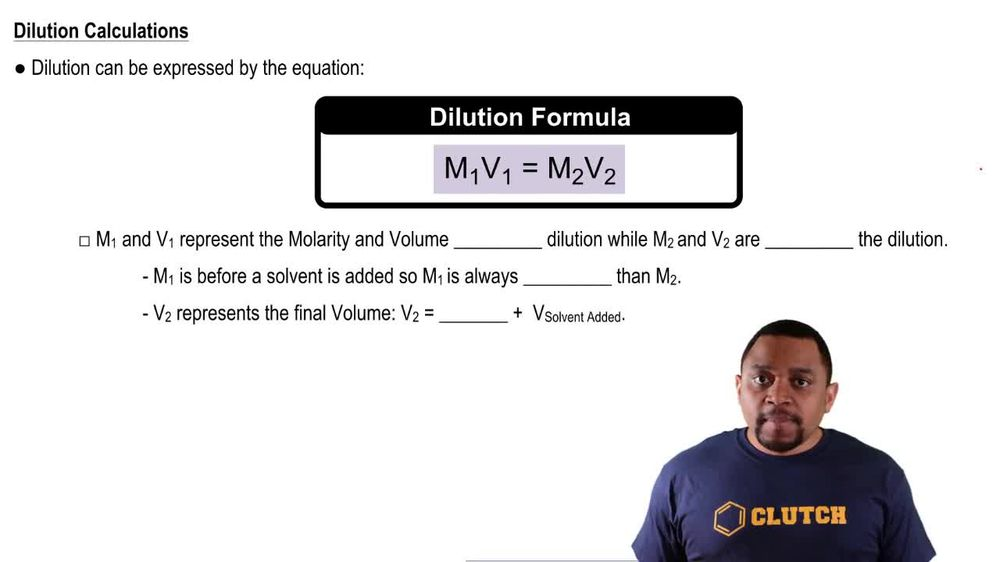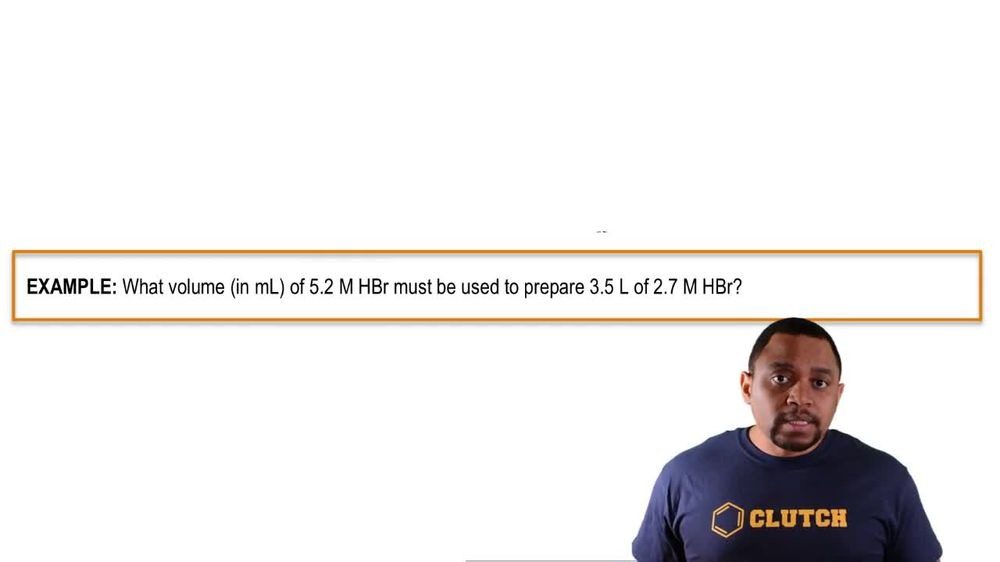Start typing, then use the up and down arrows to select an option from the list.
1. 6. Chemical Quantities & Aqueous Reactions2. Dilutions# Dilutions Example 1

by Jules Bruno
98 views
0
in this example question. It says, if each sphere represents a mole of salute from the images provided below, arrange the solutions from least concentrated to most concentrated. Alright, so least concentrated is the same thing as saying the lowest polarity most concentrated means we have the highest polarity. Now remember, Polarity itself represents moles of solute divided by leaders of solution. So if we take a look here for a a has in it 12345 Sears. So that would be five moles of solute divided by one leader of solutions. So that'd be five Mueller for B b is 123 spheres. So that's three moles of solute over to leaders of solutions that be 1.5 Mueller and then finally see, we have 123456 spheres. So six moles divided by three leaders of solution. So that's two more so arranging it from the lowest polarity toe highest polarity, we're going to say the order would be be then see, and then finally a would have the highest polarity